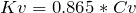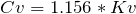# Flow Coefficient, Cv, to Flow Factor, Kv, Converter

Valve Flow Coefficient (Cv) and Flow Factor (Kv) Summaries and Conversion

Summary

Flow coefficient, Cv, and Flow Factor, Kv, are values used to specify the hydraulic capacity of a control valve.

Flow coefficient is an imperial measurement and is defined as: the flow of water through a valve at 60°F in US gallons/minute (gpm) at a pressure drop of 1lb/in².

Flow factor is the metric equivalent of flow coefficient and is defined as: the flow of water in metres cubed per hour (m³/hr) at a pressure drop of bar.

This should not be confused with Discharge Coefficient which is a non-dimensional factor of a valve used to calculate flow that discharges to the environment.

Convert Flow Coefficient to Flow Factor

 Convert flow coefficient in US gpm.psi to flow factor in (m³/hr).bar: Formula:Cv = flow coefficient Cv Result: (m³/hr).bar

Convert Flow Factor to Flow Coefficient

 Convert flow factor in (m³/hr).bar to flow coefficient in US gpm.psi: Formula:Cv = flow coefficient Cv Result: US gpm.psi